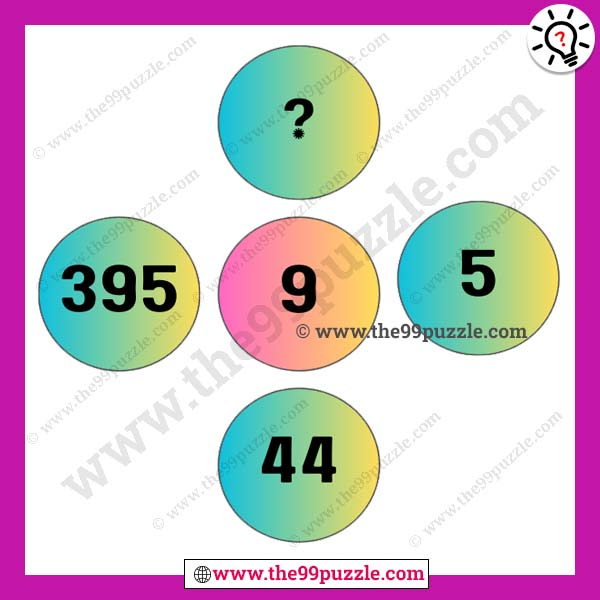# Interesting and fun circle puzzle with answer – Puzz290

This interesting and fun circle puzzle is viral on many social platforms. These types of amazing math puzzles you will find on this website. If you are a puzzle lover you also like our brain teasers puzzles question. In this math picture, you will see some numbers in the circle. You have to find out the mathematical tricks and replace the question mark. If you can practice these every day, you can solve this problem very quickly in any exam.###### Explanation:

9×5 = 45-1 = 44

9×44 = 396-1 = 395

9×395 = 3555-1 = 3554